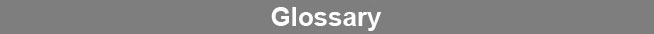Select PageProbability

Probability is the likelihood that the difference between two or more groups of the same parameter is due to random error alone. Probabilities are expressed as a decimal value between 0 and 1. A high probability indicates that there is no significant difference between the parameters, while a low probability indicates a significant difference. Probabilities in STATLIA MATRIX’s reports are the probabilities of no significant difference between a parameter in one or more assay runs and the corresponding parameter from the reference assays. Probability limits define the probability values below which the parameter being tested is considered significantly different from the reference assays.www.brendan.com